# JP4337186B2 - Image information conversion apparatus, image information conversion method, learning apparatus, and learning method - Google Patents

Image information conversion apparatus, image information conversion method, learning apparatus, and learning method Download PDF

## Info

Publication number
JP4337186B2
JP4337186B2 JP30948499A JP30948499A JP4337186B2 JP 4337186 B2 JP4337186 B2 JP 4337186B2 JP 30948499 A JP30948499 A JP 30948499A JP 30948499 A JP30948499 A JP 30948499A JP 4337186 B2 JP4337186 B2 JP 4337186B2
Authority
JP
Japan
Prior art keywords
value
output
statistical data
pixel
image signal
Prior art date
Legal status (The legal status is an assumption and is not a legal conclusion. Google has not performed a legal analysis and makes no representation as to the accuracy of the status listed.)
Expired - Fee Related
Application number
JP30948499A
Other languages
Japanese (ja)
Other versions
JP2000200349A (en
Inventor

Original Assignee
ソニー株式会社
Priority date (The priority date is an assumption and is not a legal conclusion. Google has not performed a legal analysis and makes no representation as to the accuracy of the date listed.)
Filing date
Publication date
Priority to JP30889998 priority Critical
Priority to JP10-308899 priority
Application filed by ソニー株式会社 filed Critical ソニー株式会社
Priority to JP30948499A priority patent/JP4337186B2/en
Publication of JP2000200349A publication Critical patent/JP2000200349A/en
Application granted granted Critical
Publication of JP4337186B2 publication Critical patent/JP4337186B2/en
Anticipated expiration legal-status Critical
Expired - Fee Related legal-status Critical Current

• 238000006243 chemical reactions Methods 0.000 title claims description 14
• 238000000605 extraction Methods 0.000 claims description 45
• 230000000875 corresponding Effects 0.000 claims description 17
• 230000015556 catabolic process Effects 0.000 claims description 14
• 230000004059 degradation Effects 0.000 claims description 14
• 238000006731 degradation reactions Methods 0.000 claims description 14
• 230000007274 generation of a signal involved in cell-cell signaling Effects 0.000 claims description 11
• 230000000593 degrading Effects 0.000 claims description 4
• 238000000034 methods Methods 0.000 description 19
• 238000004364 calculation methods Methods 0.000 description 16
• 230000003044 adaptive Effects 0.000 description 10
• 238000010586 diagrams Methods 0.000 description 10
• 230000015654 memory Effects 0.000 description 9
• 230000004301 light adaptation Effects 0.000 description 7
• 239000000284 extracts Substances 0.000 description 5
• 230000005540 biological transmission Effects 0.000 description 2
• 230000011218 segmentation Effects 0.000 description 2
• 230000001276 controlling effects Effects 0.000 description 1
• 230000000694 effects Effects 0.000 description 1
• 239000011159 matrix materials Substances 0.000 description 1
• 239000000203 mixtures Substances 0.000 description 1
• 230000004048 modification Effects 0.000 description 1
• 238000006011 modification reactions Methods 0.000 description 1
• 230000002123 temporal effects Effects 0.000 description 1

## Images

•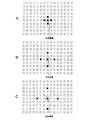•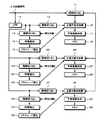•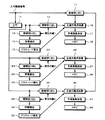•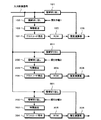•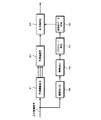•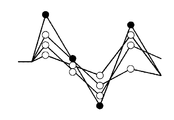•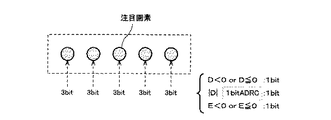•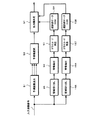••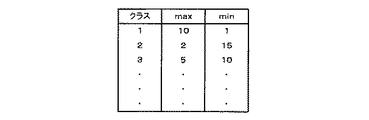## Description


BACKGROUND OF THE INVENTION
The present invention relates to an image information conversion device, an image information conversion method, a learning device, and a learning method for improving blurring of an input image signal.

[Prior art]
In recent years, processing for improving image quality by improving blur using class classification adaptive processing has been researched and developed. The class classification adaptive process performs class classification according to the signal level distribution of the input image signal, stores the prediction coefficient value obtained by learning in advance for each class in a predetermined storage unit, and weights using the prediction coefficient value This is a process of outputting an optimum estimated value as the pixel value of the target pixel by the addition formula.

In this case, in order to obtain data necessary for classifying the target pixel, data necessary for class taps arranged around the target pixel and calculation for estimating the pixel value of the target pixel If the prediction taps arranged around the pixel of interest are adaptively arranged, i.e., at the optimum thinning interval for each pixel of interest, control is not performed on such a thinning interval. Compared to this, the image quality can be greatly improved. However, such control has not been realized.

[Problems to be solved by the invention]
Accordingly, an object of the present invention is to provide an image information conversion device, an image information conversion method, a learning device, and a learning method capable of improving the degree of image quality improvement when performing image quality improvement using class classification adaptation processing. It is to provide.

[Means for Solving the Problems]
The invention according to claim 1 is a prediction image signal generation unit that generates prediction pixel values from images obtained by thinning the input image signal at a plurality of different thinning intervals, respectively.
Optimal pixel value output means for selectively outputting one of a maximum value and a minimum value of a plurality of prediction pixel values output from the prediction image signal generation means based on the characteristics of the input image signal.,
The optimum pixel value output means is
Statistical data class tap extraction means for outputting pixel values at predetermined positions specified around the pixel of interest from the input image signal as statistical data class taps;
A statistical data class code generating means for generating a statistical data class code based on the output of the statistical data class tap extracting means;
Statistical data storage means for storing statistical data in advance and outputting statistical data corresponding to the output of the statistical data class code generating means;
Output value determining means for outputting an optimum value from the maximum value and the minimum value based on the output of the statistical data storage means;This is an image information conversion device.

Claim4According to the invention, a predicted image signal generation step for generating a predicted pixel value from an image obtained by thinning an input image signal at a plurality of different thinning intervals;
One of the maximum value and the minimum value among the plurality of prediction pixel values generated by the prediction image signal generation step is selectively output based on the characteristics of the input image signal.Optimal pixel value outputWith steps,
The optimal pixel value output step is
A statistical data class tap extraction step for outputting a pixel value at a predetermined position specified around the pixel of interest from the input image signal as a statistical data class tap;
A class code generation step for statistical data that generates a class code for statistical data based on the output of the class tap extraction step for statistical data;
A statistical data output step of outputting statistical data corresponding to the statistical data class code generated by the statistical data class code generation step from the statistical data stored in advance;
Based on the output of the statistical data output step, an output value determination step for outputting an optimum value from the maximum value and the minimum value is provided.This is an image information conversion method characterized by the above.

Claim5According to the present invention, signal degradation processing means for degrading an input image signal,
Predicted image signal generating means for generating predicted pixel values, respectively, from images obtained by thinning images output from the signal degradation processing means at different thinning intervals;
An optimum pixel value output means for selectively outputting one of a maximum value and a minimum value among a plurality of prediction pixel values output from the prediction image signal generation means with reference to the input image signal;
Represents the number of pixels that satisfy a predetermined condition among the outputs of the optimum pixel value output means, classifies whether the pixel is the maximum value or the minimum value, and the characteristics of the image output from the signal degradation processing means And a counting means for counting corresponding to the output of the means for generating information.

Claim11The invention includes a signal degradation processing step for degrading an input image signal,
A predicted image signal generating step for generating predicted pixel values, respectively, from images obtained by thinning out images generated by the signal degradation processing step at different types of thinning intervals;
An optimum pixel value output step for selectively outputting one of a maximum value and a minimum value among a plurality of prediction pixel values generated by the prediction image signal generation step with reference to the input image signal;
Expresses the number of pixels that satisfy a predetermined condition among the results of the optimal pixel value output step, classifies whether the pixel is the maximum value or the minimum value, and the characteristics of the image generated by the signal degradation processing step And a step of counting corresponding to the output of the step of generating the information to be performed.

According to the invention as described above, the optimum one among the results of the class classification adaptive processing based on a plurality of types of class tap structures having different thinning intervals is selected as the output image signal.

DETAILED DESCRIPTION OF THE INVENTION
Hereinafter, an embodiment of the present invention will be described. The class classification adaptive process performs class classification according to the feature data of the input image signal such as the signal level distribution of the input image signal, and stores the prediction coefficient value obtained by learning in advance for each class in a predetermined storage unit. In this process, the optimum estimated value is output as the pixel value of the target pixel by the weighted addition formula using the prediction coefficient value.

Class taps are arranged on the input image signal in order to grasp the signal level distribution of the input image signal and perform classification. The class tap is a pixel value extracted from a predetermined position of the input image signal as data for calculating a feature expressing the signal level distribution of the input image signal. For example, the class tap is arranged on the frame including the pixel of interest and on the frames before and after it. An example of the class tap structure on the frame including the target pixel is shown in FIG. Here, in FIG. 1A, class taps are arranged at pixel positions adjacent to the thinning interval 1, that is, vertically / horizontally with respect to the target pixel. That is, when the thinning interval is 1, thinning is not performed. In FIG. 1B, the class tap is arranged at the second pixel position vertically / horizontally with respect to the thinning interval 2, that is, the target pixel. Further, in FIG. 1C, a class tap is arranged at a thinning interval 3, that is, at a third pixel position vertically / horizontally with respect to the target pixel. Similarly, a class tap arrangement in which the thinning interval is further widened, such as the thinning intervals 4 and 5 can be used. In a blurred image, it is different in which decimation interval is optimal for class classification according to the extent of the blur range.

Prior to the description of an embodiment of the present invention, a class tap arrangement set under a plurality of types of thinning amounts has already been proposed for improving the image quality of blurred images by class classification adaptive processing. The configuration will be described. First, FIG. 2 shows an example of a configuration related to learning, that is, processing for obtaining a prediction coefficient. A predetermined input image signal (referred to as a teacher signal) that does not include blur is supplied to an LPF (Low Pass Filter) circuit 10 and an area extraction circuit 11. The LPF circuit 10 performs an LPF process on the input image signal to generate a deteriorated (blurred) image signal. The deteriorated image signal is converted into the region extraction circuits 12 and 13, the region extraction circuits 22 and 23, and the region This is supplied to the clipping circuits 32 and 33.

The region cutout circuit 12 cuts out a predetermined range of pixel regions from the degraded image signal as a prediction tap, and supplies the cutout region to the normal equation addition circuit 16. Further, the region cutout circuit 13 cuts out a predetermined range of pixel regions as class taps under the class tap arrangement with the thinning interval 1 as shown in FIG. 1A and supplies the cut out region to the feature extraction circuit 14. The feature extraction circuit 14 extracts the degraded feature of the image signal based on the output of the region cutout circuit 13 and supplies the extracted feature to the class code generation circuit 15. The class code generation circuit 15 generates a class code based on the output of the feature extraction circuit 14 and supplies the generated class code to the normal equation addition circuit 16.

On the other hand, the region cutout circuit 11 cuts out a predetermined pixel region from the teacher signal and supplies the cutout region to the normal equation addition circuit 16. The normal equation adding circuit 16 performs a predetermined calculation process based on the output of the area cutout circuit 11, the output of the area cutout circuit 12, and the output of the class code generation circuit 15, and calculates a prediction coefficient for each class. Data related to the normal equation is generated, and the generated data is supplied to the prediction coefficient determination circuit 17. The prediction coefficient determination circuit 17 calculates a prediction coefficient by performing a calculation process for solving a normal equation based on the supplied data, and supplies the calculated prediction coefficient to the memory 18. The memory 18 stores the supplied prediction coefficient.

The region segmentation circuit 23, feature extraction circuit 24, class code generation circuit 25, normal equation addition circuit 26, prediction coefficient determination circuit 27, and memory 28 are the region segmentation circuit 13, feature extraction circuit 14, class code described above, respectively. This is similar to the generation circuit 15, normal equation addition circuit 16, prediction coefficient determination circuit 17, and memory 18. However, the region cutout circuit 23 performs region cutout under the class tap arrangement with the thinning interval 2 as shown in FIG. 1B.

In addition, the region extraction circuit 33, the feature extraction circuit 34, the class code generation circuit 35, the normal equation addition circuit 36, the prediction coefficient determination circuit 37, and the memory 38 are respectively the region extraction circuit 13, the feature extraction circuit 14, The class code generation circuit 15, the normal equation addition circuit 16, the prediction coefficient determination circuit 17, and the memory 18 are the same. However, the region cutout circuit 33 performs region cutout under a class tap arrangement with a thinning interval 3 as shown in FIG. 1C.

Here, the calculation related to the calculation of the prediction coefficient will be described in more detail. As will be described later with reference to FIG. 4, the predicted image signal by the class classification adaptive processing is based on a prediction tap extracted from a predetermined pixel position of the input image signal and a prediction coefficient obtained by learning. The pixel value y is sequentially predicted and generated according to the equation (1).

y = w1X1+ W2X2+ ... + wnXn      (1)
Where x1, ..., xnIs each prediction tap, w1, ..., wnAre each prediction coefficient.

The normal equation addition circuits 16, 26, and 36 are based on the prediction tap supplied from the region cutout circuits 12, 22, and 23, the class code supplied from the class code generation circuits 15, 25, and 35, and the teacher signal, respectively. The prediction coefficient w1, ..., wnData necessary for solving a normal equation with the solution is calculated. Then, the prediction coefficient determination circuits 17, 27, and 37 calculate the prediction coefficient by performing a calculation process for solving the normal equation based on the supplied data.

The normal equation will be described. In the above equation (1), before learning, the prediction coefficient w1, ..., wnIs an undetermined coefficient. Learning is performed by inputting a plurality of teacher signals for each class. When the number of types of teacher signals is expressed as m, the following equation (2) is set from equation (1).

yk= W1Xk1+ W2Xk2+ ... + wnXkn      (2)
(K = 1, 2,..., M)
If m> n, prediction coefficient w1, ..., wnIs not uniquely determined, the element e of the error vector ekIs defined by the following equation (3), and the prediction coefficient is determined so as to minimize the error vector e defined by equation (4). That is, the prediction coefficient is uniquely determined by a so-called least square method.

ek= Yk-{W1Xk1+ W2Xk2+ ... + wnXkn} (3)
(K = 1, 2, ... m)

[Expression 1]

E in equation (4)2As a practical calculation method for obtaining the prediction coefficient that minimizes2Prediction coefficient wi(i = 1, 2...) is partially differentiated (formula (5)), and each prediction coefficient w is set so that the partial differential value becomes 0 for each value of i.iShould be determined.

[Expression 2]

From equation (5), each prediction coefficient wiA specific procedure for determining the above will be described. X as in equations (6) and (7)ji, Yi(5) can be written in the form of the determinant of equation (8).

[Equation 3]

[Expression 4]

[Equation 5]

Equation (8) is generally called a normal equation. The prediction coefficient determination circuits 17, 27, and 37 perform a calculation process for solving the normal equation based on the normal equation data according to a general matrix solving method such as a sweep-out method, and perform the prediction coefficient wiIs calculated.

FIG. 2 shows a configuration in which the prediction tap thinning interval is fixed and the class tap thinning interval is changed, but the prediction tap thinning interval may be changed together with the class tap thinning interval. Such a configuration is shown in FIG. In FIG. 3, each component is the same as that shown in FIG. However, the region cutout circuits 12, 22, and 32 cut out prediction taps having tap structures with thinning intervals 1, 2, and 3, respectively.

Next, in one embodiment of the present invention, a configuration for generating a predicted image signal for each class tap arranged under each thinning interval using the prediction coefficient obtained as described above will be described. To do. An example of such a configuration is shown in FIG. An input image signal is supplied to the area cutout circuits 101, 102, 201, 202, 301, 302. Here, the input image signal may be blurred due to band limitation in the transmission path or the like, and the display image may be blurred as it is. The region cutout circuits 101, 201, and 301 cut out pixel regions used as prediction taps from the input image signal, and supply the cutout regions to the estimation calculation units 106, 206, and 306, respectively.

On the other hand, the area extraction circuits 102, 202, and 302 extract predetermined pixel areas from the supplied image signals as class taps, and supply the extracted areas to the feature extraction circuits 103, 203, and 303, respectively. The feature extraction circuits 103, 203, and 303 extract the features of the blurred image based on the outputs of the region cutout circuits 102, 202, and 302, and supply the extracted features to the class code generation circuits 104, 204, and 304, respectively. .

The class code generation circuits 104, 204, and 304 generate class codes based on the outputs of the feature extraction circuits 103, 203, and 303, and supply the generated class codes to the ROMs 105, 205, and 305, respectively. The ROMs 105, 205, and 305 store prediction coefficients calculated as described above with reference to FIG. That is, the storage contents of the memories 18, 28, and 38 in FIG. 2 or FIG. 3 are loaded in advance in the ROMs 105, 205, and 305, respectively.

The ROMs 105, 205, and 305 output prediction coefficients corresponding to the outputs of the class code generation circuits 104, 204, and 304, respectively. The output prediction coefficients are supplied to the estimation calculation units 106, 206, and 306, respectively. The estimation calculation unit 106 calculates a linear linear combination (see Expression (1)) between a prediction coefficient supplied from the ROM 105 and a pixel value used as a prediction tap supplied from the region extraction circuit 101. Each pixel value y is estimated, and a predicted image signal as a whole of the estimated pixel value y is generated.

In the predicted image generated in this way, blurring of an image due to deterioration of an input image signal in a transmission path or the like is eliminated or reduced. In the configuration illustrated in FIG. 4, the thinning intervals in the region extraction circuits 101, 201, and 301 are constant, but the region extraction circuits 101, 201, and 301 correspond to the configuration illustrated in FIG. 3, respectively. A prediction tap having a tap structure with thinning intervals 1, 2, and 3 may be cut out.

The present invention provides an optimum prediction among a plurality of prediction values such as three types obtained by using a class tap structure with a plurality of thinning intervals such as three types as described above in the class classification adaptive processing. A value is determined, and a final output image signal is created based on the determination result.

An example of an image processing apparatus according to an embodiment of the present invention is shown in FIG. The input image signal is supplied to the predicted image generation unit 51. The predicted image generation unit 51 is configured to generate a predicted image for each thinning interval, and the configuration described above with reference to FIG. 4 can be used as the predicted image generation unit 51. When such a configuration is used, the predicted image generation unit 51 generates, for example, three predicted image signals corresponding to three types of thinning intervals, and supplies these three predicted image signals to the predicted value selection circuit 53. To do. However, the types of thinning intervals and the number of predicted image signals generated corresponding to them are not limited to three. The predicted value selection circuit 53 selects the maximum predicted value and the minimum predicted value for each pixel position from the supplied predicted values, and supplies the selected predicted value to the output value determining circuit 54.

The input image signal is supplied to the area cutout circuit 55. The region cutout circuit 55 cuts out a predetermined pixel region from the input image signal as a class tap and supplies the cutout pixel region to the feature extraction circuit 56. The feature extraction circuit 56 extracts the feature of the blurred image based on the output of the region cutout circuit 55 and supplies the extracted feature to the class code generation circuit 57. The class code generation circuit 57 generates a class code based on the output of the feature extraction circuit 56 and supplies the generated class code to the statistical data ROM 58.

The statistical data ROM 58 stores statistical data as will be described later for each class code, and supplies statistical data corresponding to the class code supplied from the class code generation circuit 57 to the predicted value determination circuit 54. The predicted value determination circuit 54 refers to the supplied statistical data, and determines the optimum one of the maximum predicted value and the minimum predicted value for each pixel position supplied from the predicted value selection circuit 53. Then, an output image signal is created based on the determination result. The processing at this time will be specifically described with reference to FIG. Here, an example of the signal waveform of the original image is indicated by a black circle. An example of the signal waveform of the predicted image signal generated by the predicted image generation unit 51 at a position corresponding to this example is indicated by three white circles. As the optimum predicted value, the white circle closest to the black circle may be selected. For this purpose, the maximum of the three predicted values is selected when the peak of the signal waveform is convex upward, and the minimum of the three predicted values is selected when the peak of the signal waveform is convex downward. A value may be selected. Further, since the level difference between the original image signal and the predicted image signal is small in the portion other than the peak, any of the three predicted values may be selected as the optimal predicted value. Considering the above situation, in one embodiment of the present invention, the predicted value selection circuit 53 selects the maximum and minimum predicted values from the three predicted values generated by the predicted image generation unit 51, and the maximum The prediction value determination circuit 54 selects an optimum output image signal from among the minimum predicted values with reference to statistical data as will be described later.

In addition, as features for classification, which are output from the feature extraction circuit 56, for example, the sign and magnitude of the first derivative and the sign of the second derivative can be used. For example, in the case of using an example of a class tap structure as shown in FIG. 7 consisting of 5 horizontal taps centered on the pixel of interest, 1 for the sign of the primary differential D (ie, the sign of D) for each pixel position. 1 bit related to the magnitude of the first derivative D (that is, a quantized value obtained by quantizing the value of | D | by 1 bit ADRC), and 1 bit related to the sign of the second derivative E (that is, the sign of E) Assign bits. Therefore, the number of bits for 5 pixels is 3 × 5 = 15 bits, and the total number of classes is 2.15= 32768 classes. You may make it thin out about 5 horizontal taps used here. The primary differential D and the secondary differential E are calculated by the following formulas (9) and (10), respectively.

In one embodiment of the present invention, it is assumed that the ADRC circuit 3 compresses the 5-pixel SD data separated by the area cut-out circuit 2 to 2 bits each. Hereafter, each compressed SD data is q1~ QFiveIs written. These pattern compressed data are supplied to the class code generation circuit 6.

D (i, j) = f (i, + j) −f (i, j−1) (9)
E (i, j)
= F (i, j + 1) + f (i, j-1) -2 * f (i, j) (10)
Here, i and j are coordinates representing the pixel position two-dimensionally, and f (i, j) is a pixel value at the pixel position. Here, the case where the class taps are arranged in two dimensions has been described as an example, but the present invention can also be applied to the case where the class taps are arranged in three dimensions (time-space). Note that 1-bit ADRC (Adaptive Dynamic Range Coding) is a process for expressing a temporal or spatial change pattern of some data with a small number of bits. When the dynamic range of | D | is DR, the bit allocation is n, the data level of | D | at each pixel position is L, and the requantization code is Q, the maximum value | D | And the minimum value min are equally divided by a designated bit length to perform requantization.

DR = MAX-MIN + 1
Q = {(L−MIN + 0.5) × 2 / DR} (11)
However, {} means a truncation process.

If the predicted value determination circuit 54 is configured to operate with reference to two types of statistical data, the optimal predicted value can be determined with higher accuracy. An example of such a configuration is shown in FIG. In FIG. 8, the same components as those in FIG. 5 are denoted by the same reference numerals. An example of such a configuration includes a first processing sequence including a region extraction circuit 55, a feature extraction circuit 56, a class code generation circuit 57, and a statistical data ROM 58 as a processing sequence related to the output of statistical data, and a region extraction circuit. 155, a feature extraction circuit 156, a class code generation circuit 157, and a second processing sequence including a statistical data ROM 158.

Here, when an ambiguous determination is made as a result of the operation of the predicted value determination circuit 54 with reference to the first statistical data output from the statistical data ROM 58 by the process performed by the first series, The optimum predicted value is determined by a control method such as controlling the predicted value determining circuit 54 to operate by referring to the second statistical data output from the statistical data ROM 158 by the processing performed by the second series. Accuracy can be improved. In addition, by providing three or more series of configurations for generating class codes, and having three or more statistical data ROMs storing statistical data corresponding to each of the three or more types of class codes generated by these, It is also possible to further improve the accuracy of determining the optimum predicted value by causing the predicted value determining circuit 54 to operate by referring to three or more types of statistical data.

Next, generation of statistical data referred to in the operation of the predicted value determination circuit 54 will be described with reference to FIG. An input image signal as a teacher signal is supplied to the LPF 61, the optimum predicted value selection circuit 64, and the residual calculation circuit 65. The LPF 61 performs an LPF process on the supplied image signal to generate a deteriorated image signal. The output of the LPF 61 is supplied to the predicted image generation unit 62 and the region cutout circuit 66.

The predicted image generation unit 62 is configured to generate a predicted image for each thinning interval. As the predicted image generation unit 62, one having the same configuration as the predicted pixel generation unit 51 in FIGS. 5 and 8 is used. . For example, three predicted image signals that are outputs of the predicted image generation unit 62 are supplied to the first predicted value selection circuit 63. The predicted value selection circuit 63 selects the maximum predicted value MAX and the maximum predicted value MIN for each pixel position from the predicted values supplied from the predicted image generation unit 62 and supplies the selected predicted value MAX to the optimal predicted value output circuit 64. .

The optimum predicted value output circuit 64 selects the output from the predicted value selection circuit 63 that has a small residual value with respect to the teacher signal, that is, the absolute value of the signal level difference, and optimizes the selected predicted value (that is, MAX or MIN). To the residual calculation circuit 65 as a predicted value. Further, the optimum predicted value output circuit 64 supplies the signal d count circuit 69 indicating whether the selected predicted value is MAX or MIN. The residual calculation circuit 65 calculates a residual based on the optimal prediction value supplied from the optimal prediction value selection circuit 64 and the teacher signal, and supplies the calculation value to the count circuit 69.

On the other hand, the region cutout circuit 66 cuts out a predetermined pixel region from the output of the LPF 61 as a class tap and supplies the cutout pixel region to the feature extraction circuit 67. The feature extraction circuit 67 extracts the features of the image by performing the same operation as the feature extraction circuit 56 in FIG. Features extracted by the feature extraction circuit 67 are supplied to the class code generation circuit 68. The class code generation circuit 68 generates a class code based on the output of the feature extraction circuit 67 and supplies the generated class code to the count circuit 69. The region cutout circuit 66, feature extraction circuit 67, and class code generation circuit 68 are the same as the region cutout circuit 55, feature extraction circuit 56, and class code generation circuit 57 in FIG.

The count circuit 69 transmits only pixels whose residuals supplied from the residual calculation circuit 65 are larger than a predetermined threshold value by the class code supplied from the class code generation circuit 68 and the signal d described above. Statistical data is generated by counting according to information whether the pixel is selected by the optimum predicted value selection circuit 64 as a maximum value or a minimum value. The generated statistical data is supplied to the memory 70 and stored therein. Here, whether or not a certain pixel is to be counted can be determined according to, for example, whether or not the residual relating to the pixel is larger than a predetermined threshold value. An example of statistical data is shown in FIG. In FIG. 10, when the class code indicates class 1, the pixel is the pixel when the maximum value is selected by the optimum prediction value selection circuit 64, and the residual between the pixel and the teacher signal is larger than the threshold value. When the maximum number is selected by the optimum predicted value output circuit 64 and the absolute value of the residual between the pixel and the teacher signal is greater than the threshold value, the count number is 10 1 is shown.

In addition, when the class code indicates class 2, it is indicated that the count number of the pixel selected as the maximum value / minimum value and having a large residual between the pixel and the teacher signal is 2/15. ing. Similarly, when the class code indicates class 3, it is indicated that the count number of the pixel selected as the maximum value / minimum value and having a large residual between the pixel and the teacher signal is 5/10. Has been.

In this way, the degree of contribution to the improvement of image quality in relation to conditions such as thinning intervals by obtaining statistical data by limiting only residual pixels, that is, counting only pixels whose residual is larger than a certain level. Are excluded, and only the part that contributes to the improvement of image quality is reflected in the statistical data.

Further, the portion reflected in the statistical data may be limited by paying attention to an amount other than the residual for each pixel between the optimal prediction value and the teacher signal. For example, a configuration in which the portion reflected in the statistical data is limited by paying attention to the size of the dynamic range DR or the differential value among a plurality of predicted values for each thinning interval is also possible. Depending on what kind of restrictions are imposed at this time, a plurality of types of statistical data can be obtained. In this way, it is possible to generate a plurality of types of statistical data used in the configuration described above with reference to FIG. Further, the processing in the region extraction circuit 55, the region extraction circuit 155, the feature extraction circuit 56, and the feature extraction circuit 156 may be changed.

The statistical data stored in the memory 70 is loaded into the statistical data ROM 58 shown in FIGS. The prediction value determination circuit 54 in FIGS. 5 and 8 is suitable for the one having the largest count value in the statistical data among the maximum pixel value and the minimum pixel value supplied from the prediction value selection circuit 53. The pixel value is determined. However, if the count values for the maximum pixel value and the minimum pixel value in the statistical data are in conflict with each other, if a suitable prediction pixel value is determined with reference to the statistical data, the reliability of the determination result It is thought that the nature is low. In such a case, if the determination result is incorrect, there is a possibility that a significant deterioration in image quality may occur.

In view of this, when the count value for the maximum pixel value and the minimum pixel value in the statistical data is in competition with each other, the following process is performed, so that the prediction value determination circuit 54 can perform a suitable prediction. The reliability of the determination of the pixel value can be ensured. {Circle around (1)} In the configuration shown in FIG. 8, statistical data other than the statistical data is referred to in the operation of the predicted value determination circuit 54. (2) The average value of the maximum pixel value and the minimum pixel value is output from the predicted value determination circuit 54. Here, (1) and (2) are both examples and are not limited to these.

In addition, when the count value for the maximum pixel value and the minimum pixel value in the statistical data is in competition with each other, for example, it can be determined that the following equation (12) holds.

a / (ab) <threshould (12)
Here, a represents the higher frequency, and b represents the lower frequency. Threshould is a predetermined threshold value.

The present invention is not limited to the above-described embodiment of the present invention, and various modifications and applications can be made without departing from the gist of the present invention.

【The invention's effect】
As described above, according to the present invention, an optimum one is obtained by referring to statistical data from among the results of class classification adaptation processing based on a plurality of types of class tap structures and / or prediction tap structures having different thinning intervals. The selected image signal is used as an output image signal.

For this reason, for each pixel position in the input image signal, using the result of class classification adaptation processing under a thinning interval that is more compatible with the degree of image degradation at the pixel position, features in the image, etc. An output image signal can be generated.

In particular, according to the present invention, since an optimum thinning interval can be selected for each pixel, a larger image quality improvement can be realized as compared with the case where class classification adaptive processing is performed on the entire image signal under a certain thinning interval. be able to.

In addition, by detecting the maximum value and minimum value from the results of class classification adaptation processing under different thinning intervals, and selecting the optimum value from the detected maximum value and minimum value Compared with the case where the optimum one is selected from all the results of class classification adaptation processing (for example, three predicted image signals) under different thinning intervals, it is easier and more accurate especially near the peak of the signal waveform. The optimal prediction image signal can be selected.

Furthermore, in the process of obtaining the statistical data, more accurate statistical data can be obtained for improving the image quality by taking into account predetermined conditions related to the characteristics of the image signal.

Further, if a configuration using a plurality of types of statistical data is used, a greater improvement in image quality can be realized.
[Brief description of the drawings]
FIG. 1 is a schematic diagram illustrating an example of a class tap structure at each thinning interval.
FIG. 2 is a block diagram illustrating an example of a configuration related to learning in a class classification adaptation process.
FIG. 3 is a block diagram showing another example of a configuration related to learning in the class classification adaptation process.
FIG. 4 is a block diagram illustrating an example of a configuration relating to prediction estimation in a class classification adaptive process.
FIG. 5 is a block diagram showing an example of a configuration related to prediction estimation in one embodiment of the present invention.
FIG. 6 is a schematic diagram illustrating an example of a signal waveform of an original image signal and a signal waveform of a predicted image signal.
FIG. 7 is a schematic diagram for explaining an example of class classification when referring to statistical data;
FIG. 8 is a block diagram showing another example of the configuration according to the embodiment of the present invention.
FIG. 9 is a block diagram showing an example of a configuration for obtaining statistical data according to an embodiment of the present invention.
FIG. 10 is a schematic diagram illustrating an example of statistical data.
[Explanation of symbols]
51 ... Predictive image output unit, 53 ... Predictive value selection circuit, 54 ... Output value determination circuit, 58 ... Statistical data ROM

## Claims (11)

1. Predicted image signal generation means for generating predicted pixel values from images obtained by thinning input image signals at different thinning intervals;
Optimal pixel value output means for selectively outputting one of a maximum value and a minimum value among a plurality of prediction pixel values output from the prediction image signal generation means based on the characteristics of the input image signal ;
The optimum pixel value output means includes:
Statistical data class tap extraction means for outputting, as the statistical data class tap, a pixel value at a predetermined position designated around the pixel of interest from the input image signal;
A statistical data class code generating means for generating a statistical data class code based on the output of the statistical data class tap extracting means;
Statistical data storage means for storing statistical data in advance and outputting statistical data corresponding to the output of the statistical data class code generating means;
On the basis of the output of the statistical data storing means, from among the maximum value and the minimum value, the image information converting apparatus according to claim Rukoto an output value determination unit for outputting an optimum value.
2. In claim 1,
The above-described different types of thinning intervals are
What is claimed is: 1. An image information conversion apparatus including a device corresponding to a case where thinning is not performed.
3. In claim 1,
An image information conversion apparatus using two or more kinds of statistical data.
4. A predicted image signal generating step for generating predicted pixel values from images obtained by thinning the input image signal at a plurality of different thinning intervals;
An optimal pixel value output step for selectively outputting one of a maximum value and a minimum value among a plurality of prediction pixel values generated by the prediction image signal generation step based on the characteristics of the input image signal ;
The optimal pixel value output step includes:
A statistical data class tap extraction step for outputting, as a statistical data class tap, a pixel value at a predetermined position designated around the pixel of interest from the input image signal;
A statistical data class code generation step for generating a statistical data class code based on the output of the statistical data class tap extraction step;
A statistical data output step of outputting statistical data corresponding to the statistical data class code generated by the statistical data class code generating step from the statistical data stored in advance;
On the basis of the output of the statistical data outputting step, the image information conversion method for from among the maximum and minimum values, characterized in Rukoto and an output value determination step of outputting an optimum value.
Predicted image signal generating means for generating predicted pixel values, respectively, from images obtained by thinning out images output from the signal degradation processing means at different thinning intervals;
An optimum pixel value output means for selectively outputting one of a maximum value and a minimum value among a plurality of prediction pixel values output from the prediction image signal generation means with reference to the input image signal;
Among the outputs of the optimal pixel value output means, the number of those satisfying a predetermined condition is classified into the maximum value or the minimum value of the pixel, and the image output from the signal deterioration processing means. A learning apparatus comprising: counting means for counting corresponding to the output of the means for generating information representing the feature.
6. In claim 5 ,
The optimum pixel value output means includes:
Among the plurality of predicted pixel values output from the predicted image signal generating means, the one having a smaller residual with the pixel value at the corresponding position in the input image is selectively selected from among the maximum and minimum values among the plurality of predicted pixel values. A learning device characterized by outputting.
7. In claim 5 ,
Means for generating information representing the characteristics of the image output from the signal degradation processing means,
A class tap extracting means for outputting a pixel value at a predetermined position designated around the pixel of interest from the image output from the signal degradation processing means as a statistical data class tap;
A learning apparatus comprising class code generation means for generating a class code based on an output of the class tap extraction means.
8. In claim 5 ,
The predetermined condition is as follows:
An image information conversion apparatus, wherein a residual between the optimum predicted pixel value and a pixel value at a corresponding position in the predetermined image signal is larger than a predetermined threshold value.
9. In claim 5 ,
The predetermined condition is as follows:
An image information conversion apparatus characterized by being related to a dynamic range value when expressing a plurality of predicted pixel values.
10. In claim 5 ,
The predetermined condition is as follows:
An image information conversion apparatus characterized by being related to a spatial differential value of the optimum predicted pixel value.
11. A signal degradation processing step for degrading the input image signal;
A predicted image signal generating step for generating predicted pixel values, respectively, from images obtained by thinning out the images generated by the signal degradation processing step at different thinning intervals;
An optimum pixel value output step for selectively outputting one of a maximum value and a minimum value among a plurality of prediction pixel values generated by the prediction image signal generation step with reference to the input image signal;
Among the results of the optimal pixel value output step, the number of those satisfying a predetermined condition is classified as to whether the pixel is the maximum value or the minimum value, and the image generated by the signal degradation processing step. And a step of counting corresponding to the output of the step of generating information representing the feature.
JP30948499A 1998-10-29 1999-10-29 Image information conversion apparatus, image information conversion method, learning apparatus, and learning method Expired - Fee Related JP4337186B2 (en)

## Priority Applications (3)

Application Number Priority Date Filing Date Title
JP30889998 1998-10-29
JP10-308899 1998-10-29
JP30948499A JP4337186B2 (en) 1998-10-29 1999-10-29 Image information conversion apparatus, image information conversion method, learning apparatus, and learning method

## Applications Claiming Priority (1)

Application Number Priority Date Filing Date Title
JP30948499A JP4337186B2 (en) 1998-10-29 1999-10-29 Image information conversion apparatus, image information conversion method, learning apparatus, and learning method

## Publications (2)

Publication Number Publication Date
JP2000200349A JP2000200349A (en) 2000-07-18
JP4337186B2 true JP4337186B2 (en) 2009-09-30

# Family

## Family Applications (1)

Application Number Title Priority Date Filing Date
JP30948499A Expired - Fee Related JP4337186B2 (en) 1998-10-29 1999-10-29 Image information conversion apparatus, image information conversion method, learning apparatus, and learning method

## Country Status (1)

JP (1) JP4337186B2 (en)

## Families Citing this family (4)

* Cited by examiner, † Cited by third party
Publication number Priority date Publication date Assignee Title
JP4645867B2 (en) * 2000-08-02 2011-03-09 ソニー株式会社 Digital signal processing method, learning method, device thereof, and program storage medium
JP4311258B2 (en) 2004-04-02 2009-08-12 ソニー株式会社 Coefficient data generating apparatus and generating method, coefficient seed data generating apparatus and generating method, information signal processing apparatus, program, and recording medium recording the same
JP4604622B2 (en) * 2004-09-17 2011-01-05 ソニー株式会社 Image display apparatus and control method
JP4825748B2 (en) * 2007-07-13 2011-11-30 株式会社モルフォ Image data processing method and imaging apparatus

## Also Published As

Publication number Publication date
JP2000200349A (en) 2000-07-18

## Similar Documents

Publication Publication Date Title
KR102103984B1 (en) Method and apparatus processing a depth image
US5469216A (en) Apparatus and method for processing a digital video signal to produce interpolated data
JP3621152B2 (en) Feature point identification apparatus and method
JP3196906B2 (en) Image signal encoding device
KR100360206B1 (en) Image signal converter
KR101023451B1 (en) Image processing device and method, learning device and method, recording medium, and program
US6301385B1 (en) Method and apparatus for segmenting images prior to coding
CN101322403B (en) Auto-regressive method and filter for denoising images and videos
US5136374A (en) Geometric vector quantization
KR100563552B1 (en) Method and apparatus for performing hierarchical motion estimation using nonlinear pyramid
US7532762B2 (en) Image coding/decoding method, image coding apparatus and image decoding apparatus for obtaining decoded images having small distortion in DCT based standard coding/decoding
KR100289592B1 (en) Layer encoding and decoding device for digital video signal
EP0614318B1 (en) Video encoder and decoder
DE69725765T2 (en) Image decoder and method for image decoding
US5701367A (en) Image data coding system for controlling amounts of code data for character image and natural image regions
EP1555804A2 (en) Image processing apparatus, image processing program and storage medium
Sneyers et al. FLIF: Free lossless image format based on MANIAC compression
KR20010033797A (en) Apparatus and method for performing scalable hierarchical motion estimation
EP0794513A1 (en) Method and enhancing image using quantized mean-separate histogram equalization and a circuit therefor
JP5237969B2 (en) Contour reduction in digital images
EP0620686B1 (en) Fuzzy-controlled coding method and apparatus therefor
EP0368139A2 (en) Method for coding residual error pictures
KR20000076522A (en) Motion estimation using orthogonal transform-domain block matching
US7667711B2 (en) Image processing system, a method thereof, and a recording medium thereof
KR100934397B1 (en) Apparatus and method for generating coefficient data, apparatus and method for processing information signal using same, apparatus and method for generating coefficient data for use therein, and information providing medium

## Legal Events

Date Code Title Description
A621 Written request for application examination

Free format text: JAPANESE INTERMEDIATE CODE: A621

Effective date: 20060314

A131 Notification of reasons for refusal

Free format text: JAPANESE INTERMEDIATE CODE: A131

Effective date: 20090303

A521 Written amendment

Free format text: JAPANESE INTERMEDIATE CODE: A523

Effective date: 20090507

TRDD Decision of grant or rejection written
A01 Written decision to grant a patent or to grant a registration (utility model)

Free format text: JAPANESE INTERMEDIATE CODE: A01

Effective date: 20090609

A01 Written decision to grant a patent or to grant a registration (utility model)

Free format text: JAPANESE INTERMEDIATE CODE: A01

A61 First payment of annual fees (during grant procedure)

Free format text: JAPANESE INTERMEDIATE CODE: A61

Effective date: 20090622

FPAY Renewal fee payment (event date is renewal date of database)

Free format text: PAYMENT UNTIL: 20120710

Year of fee payment: 3

FPAY Renewal fee payment (event date is renewal date of database)

Free format text: PAYMENT UNTIL: 20130710

Year of fee payment: 4

R250 Receipt of annual fees

Free format text: JAPANESE INTERMEDIATE CODE: R250

R250 Receipt of annual fees

Free format text: JAPANESE INTERMEDIATE CODE: R250

R250 Receipt of annual fees

Free format text: JAPANESE INTERMEDIATE CODE: R250

LAPS Cancellation because of no payment of annual fees